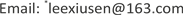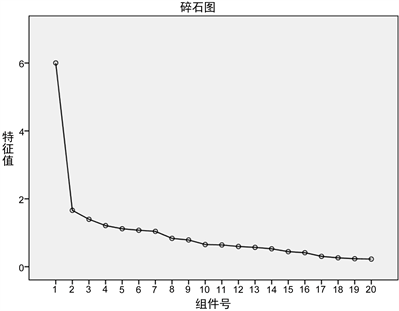﻿ 基于因子分析和有序逻辑回归的大学生数学成绩与平时学习情况的相关性分析 Relevance Analysis of Mathematics Achievement of College Students and Their Learning Situation in Peacetime Based on Factor Analysis and Ordered Logic Regression

Vol. 08  No. 07 ( 2019 ), Article ID: 31318 , 4 pages
10.12677/ASS.2019.87167

Relevance Analysis of Mathematics Achievement of College Students and Their Learning Situation in Peacetime Based on Factor Analysis and Ordered Logic Regression

Haiqin Li, Xiusen Li*, Yue Sun

School of Mathematics and Statistics, Shandong University of Technology, Zibo ShandongReceived: Jun. 28th, 2019; accepted: Jul. 11th, 2019; published: Jul. 18th, 2019ABSTRACT

We conducted a questionnaire survey on more than 300 students from the School of Economics, Shandong University of Technology. The questionnaire was designed from four general aspects: pre-class preparation, classroom learning, after-class learning and homework, and pre-examination review. Finally, 259 valid data were obtained. Each option of the questionnaire was assigned a number of values, and factor analysis was carried out by introducing SPSS. Then the final results were divided into four levels: excellent, good, pass and fail. The correlation between students’ math scores and their normal learning situation was analyzed and studied by orderly logistic regression analysis.

Keywords:University Mathematics, Peacetime Learning Situation, Factor Analysis, Ordered Logic Regression Analysis1. 引言

2. 数学成绩与平时学习情况的因子分析Figure 1. Scree plot

F1 = 0.165 * 文理科 + 0.119 * 高考数学成绩 + 0.243 * 听课认真程度 − 0.030 * 学习时间 − 0.037 *做往届试题 − 0.190 * 参加模拟考试 − 0.051 * 整理笔记 − 0.005 * 错题整理 − 0.150 * 自觉做作业 − 0.035 * 保存作业 − 0.032 * 作业自我批改情况 + 0.070 * 作业认真情况 + 0.041 * 作业独立情况 + 0.030 * 课后复习情况 + 0.256 * 玩手机情况 + 0.275 * 回答问题跟上思路 + 0.108 * 课堂纪律遵守情况 + 0.062 * 课前预习情况 + 0.244 * 课堂内容理解程度 − 0.096 * 考研计划

3. 数学成绩与平时学习情况的有序逻辑回归分析

4. 结论

Relevance Analysis of Mathematics Achievement of College Students and Their Learning Situation in Peacetime Based on Factor Analysis and Ordered Logic Regression[J]. 社会科学前沿, 2019, 08(07): 1212-1215. https://doi.org/10.12677/ASS.2019.87167

1. 1. 张志红, 耿兴芳. 学习态度对大学生数学成绩影响的实证分析[J]. 中国大学数学报, 2009(10): 87-89.

2. 2. 张文颖, 张玉, 王冉. 大学成绩生影响因素的主成分分析[J]. 科技创新导报, 2010(20): 175.

3. 3. 孔慧华, 潘晋孝. 大学生成绩的统计分析[J]. 甘肃联合大学学报(自然科学版), 2010, 24(4): 37-40.

4. 4. 刘巧芝, 曹婧姝, 车蕙. 非智力因素对大学生学习成绩的影响[J]. 教育探索, 2009(5): 127-128.

5. NOTES

*通讯作者。